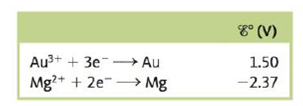# Consider a galvanic cell based on the following half-reactions: a. What is the standard potential for this cell? b. A nonstandard cell is set up at 25°C with [Mg 2+ ] = 1.00 × 10 −5 M . The cell potential is observed to be 4.01 V. Calculate [Au 3+ ] in this cell.### Chemistry: An Atoms First Approach

2nd Edition
Steven S. Zumdahl + 1 other
Publisher: Cengage Learning
ISBN: 9781305079243

#### Solutions

Chapter
Section### Chemistry: An Atoms First Approach

2nd Edition
Steven S. Zumdahl + 1 other
Publisher: Cengage Learning
ISBN: 9781305079243
Chapter 17, Problem 137CWP
Textbook Problem
5 views

## Consider a galvanic cell based on the following half-reactions:a. What is the standard potential for this cell?b. A nonstandard cell is set up at 25°C with [Mg2+] = 1.00 × 10−5 M. The cell potential is observed to be 4.01 V. Calculate [Au3+] in this cell.

(a)

Interpretation Introduction

Interpretation:

The half-reactions taking place in a galvanic cell is given. The value of standard cell potential and the concentration of Au3+ is to be calculated.

Concept introduction:

The relationship between reduction potential and standard reduction potential value and activities of species present in an electrochemical cell at a given temperature is given by the Nernst equation.

The value of Ecell is calculated using Nernst formula,

E=E°(RTnF)ln(Q)

At room temperature, the above equation specifies as,

E=E°(0.0591n)log(Q)

To determine: The value of the standard cell potential for the given cell.

The value of standard cell potential is 3.87V_ .

### Explanation of Solution

The reaction taking place at the cathode is,

Au3++3eAuE°red=1.50V

The reaction taking place at the anode is,

MgMg2++2eE°OX=2.37V

Multiply reduction half-reaction with a coefficient of 2 and oxidation half-reaction with a coefficient of 3 and then add both the reactions as,

2Au3++6e2Au3Mg3Mg2++6e

The final equation is,

2Au3++3Mg2Au+3Mg2+ </

(b)

Interpretation Introduction

Interpretation:

The half-reactions taking place in a galvanic cell is given. The value of standard cell potential and the concentration of Au3+ is to be calculated.

Concept introduction:

The relationship between reduction potential and standard reduction potential value and activities of species present in an electrochemical cell at a given temperature is given by the Nernst equation.

The value of Ecell is calculated using Nernst formula,

E=E°(RTnF)ln(Q)

At room temperature, the above equation specifies as,

E=E°(0.0591n)log(Q)

To determine: The concentration of Au3+ for the given cell.

The concentration of Au3+ in the cell is 6.02×108M_ .

### Still sussing out bartleby?

Check out a sample textbook solution.

See a sample solution

#### The Solution to Your Study Problems

Bartleby provides explanations to thousands of textbook problems written by our experts, many with advanced degrees!

Get Started

Find more solutions based on key concepts
Vitamin A supplements can help treat acne. T F

Nutrition: Concepts and Controversies - Standalone book (MindTap Course List)

A child of mass m swings in a swing supported by two chains, each of length R. If the tension in each chain at ...

Physics for Scientists and Engineers, Technology Update (No access codes included)